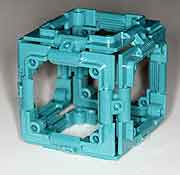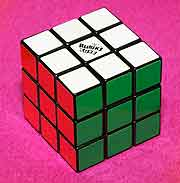JBQ's spot on the Wild Wild Web The musings of a French mathematician living in the heart of the American technology industry

The regular hexahedron
The regular hexahedron is the most common of all polyhedra. It is commonly known as the cube. It has 6 faces,which are all squares. It has 12 edges and 8 vertices.

The regular hexahedron is part of the family of 5 platonic solids, which are the most regular polyhedra. It is also part of the family of prisms (it is made of two identical regular parallel polygons joined by a row of squares).

If you join the centers of the faces of a hexahedron, you'll get an octahedron (mathematically, that means that the octahedron is the dual ofthe hexahedron). It's also worth mentioning that the regular hexahedron can tile space, and it's the only regular polyhedron with that property (mathematically, there's only one regular honeycomb, where "honeycomb" is the name for a set of polyhedra that fill 3-dimensional space).

The regular hexahedron defines three sets of symmetry axes: one set of 4 3-fold axes that join opposite vertices, one set of 6 2-fold axes that join the middle of opposite edges, and one set of 3 4-fold axes that join the middle of opposite faces.

Hexahedra occur in nature, and especially in chemistry: many ioniccrystals, including sodium chloride (i.e. table salt), have their ions arranged according to hexahedra. Other kinds of crystals follow some more complex patterns that also include hexahedra, and that's in fact the case of the diamond lattice.

The regular hexahedron is the shape that is used to build 6-sided dice.

The regular hexahedron can be built with various construction toys, like Zome, Googolplex, Polymorf, Jovo, and even Lego and K'nex - the easiest way to build a regular hexahedron with Lego bricks is to sandwich a 2x2 brick between two 2x2 plates.

The following table lists the various dimensions in a regular hexahedron, assuming that the edge length is 1:

 single edge length 1 total edge length 12 face inradius 0.5 face circumradius 0.707 polyhedron inradius 0.5 polyhedron midradius 0.707 polyhedron circumradius 0.866 single face area 1 total face area 6 volume 1

The following table is the reciprocal of the previous one, it lists the edge length that yields a value of 1 fo the different measurements in a regular hexahedron:

 1 single edge length 0.083 total edge length 2 face inradius 1.414 face circumradius 2 polyhedron inradius 1.414 polyhedron midradius 1.155 polyhedron circumradius 1 single face area 0.408 total face area 1 volume

The following table lists various angles in a regular hexahedron:

 angle between vertices (from center) 70.5 degrees angle between edges 90 degrees angle between faces 90 degrees